# SSAT Middle Level Math : How to add fractions

## Example Questions

### Example Question #31 : How To Add Fractions

Evaluate: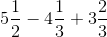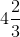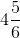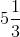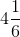Explanation:

Rewrite each as an improper fraction, then as a fraction in terms of the least common denominator, which is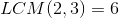: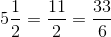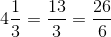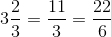Rewrite the expression, evaluate it, and rewrite the result as a mixed number: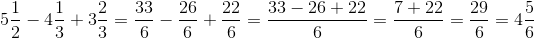### Example Question #32 : How To Add Fractions

Find the sum: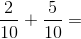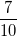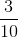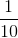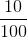Explanation:

Since the denominators of the two fractions are the same, we can simply add the numerators: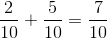### Example Question #411 : Arithmetic

Find the sum: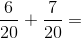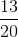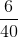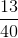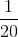Explanation:

Since the denominators of the two fractions are the same, we can simply add the numerators: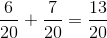### Example Question #34 : How To Add Fractions

Find the sum: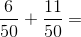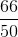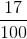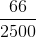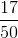Explanation:

Since the denominators of the two fractions are the same, we can simply add the numerators: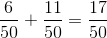### Example Question #35 : How To Add Fractions

Solve: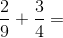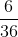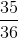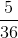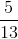Explanation:

Find the least common denominator betweenand. In this case, it is.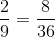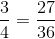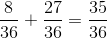### Example Question #36 : How To Add Fractions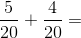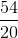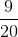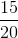Explanation:

Add the numerators and keep the denominator: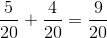Answer:### Example Question #37 : How To Add Fractions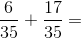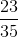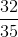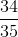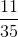Explanation:

Add the numerators and keep the denominator: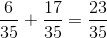Answer:### Example Question #38 : How To Add Fractions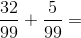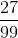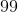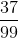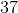Explanation:

Add the numerators and keep the denominator the same: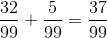Answer:### Example Question #39 : How To Add Fractions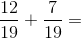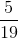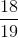Explanation:

Add the numerators and keep the denominator the same. Then, simplify: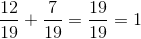Answer:### Example Question #40 : How To Add Fractions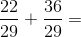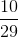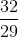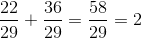Answer: